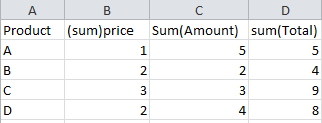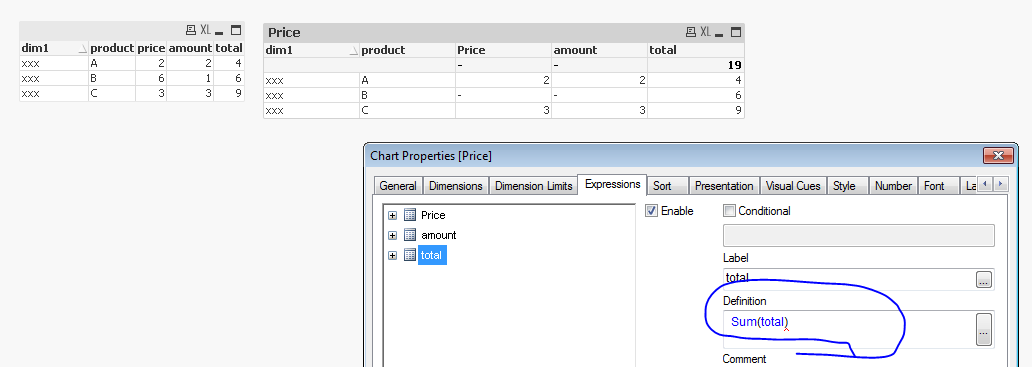# QlikView App Dev

Discussion Board for collaboration related to QlikView App Development.

Announcements
Now Live: Qlik Sense SaaS Simplified Authoring – Analytics Creation for Everyone: READ DETAILS
cancel
Showing results for
Did you mean:Not applicable

## condition based expression

from this:to this, zero values are also fine:I want to hide price and amount from product B and only show totals for the products A en C, i cant figure this out in Qlikview.
any suggestions?

Im using a pivot table with three expressions:

Amount:

(sum(amount)/count(DISTINCT Month))

Price:

(sum(total))/

(sum(amount)/count(DISTINCT Month))

total:

sum(total)

1 Solution

Accepted SolutionsMVP

Maybe something like this to show only Amount and Price for D

(sum({<Product = {D}>} amount)/count(<Product = {D}>}  DISTINCT Month))

Or

If( Match(Product,'D'),(sum(amount)/count(DISTINCT Month)), '')

[both expressions for Amount calculation in a chart with dimension Product]

8 RepliesMVP

Do you have a sample you can share with us?MVP

Maybe something like this to show only Amount and Price for D

(sum({<Product = {D}>} amount)/count(<Product = {D}>}  DISTINCT Month))

Or

If( Match(Product,'D'),(sum(amount)/count(DISTINCT Month)), '')

[both expressions for Amount calculation in a chart with dimension Product]Master II

Expression based on condition

don't put condition on total else apply condition like above link suggested.Not applicable
Author

this worked thank you, is there a way to turn zero values into white cells ?Not applicable
Author

Hi Tim Janse, if you want see just the products 'A' and 'C' add a 'Calculated Dimension' in place of 'Product' like dimension.

Try this 'Calculated Dimension':

if( Product='A' or Product='C' , Product)

With this, you will see in your Pivot Table the row values for product 'A' and 'C'.

RegardsNot applicable
Author

But if i do that i cant see the totals for product BMVP

And to keep  the threads in-sync: